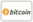Nonassociative rings with skew derivations and rings with associators in the nuclei的封面

# Nonassociative rings with skew derivations and rings with associators in the nuclei有获得代金券的资格
ISBN-13:

### 978-3-639-82411-7

ISBN-10:
3639824113
EAN:
9783639824117

We give preliminaries. In  we obtain that if R is a prime ring with a s-derivation d such that sd=ds, and d(R) ⊆ N∩L or N∩M or M∩L then R is associative or d^2=2d=0. Applying the result, we have the theorem by Posner for the prime nonassociative rings. The result  is as follows: if R is a prime ring with a skew derivation d such that ax + d(x) ∈ G for all x in R where a ∈ Z the ring of rational integers or a ∈G∩C then R is associative or d(G) = 0 Finally we generalize Kleinfeld’s result .

https://www.goldenlight-publishing.com

Chen-Te Yen

72

2016-05-24

16.80 €

Nuclei, nucleus, Nonassociative ring, commutative center, s-derivation s-invariant, d-invariant, associator ideal, extended centroid, semiprime ring, prime ring simple ring### 时事通讯